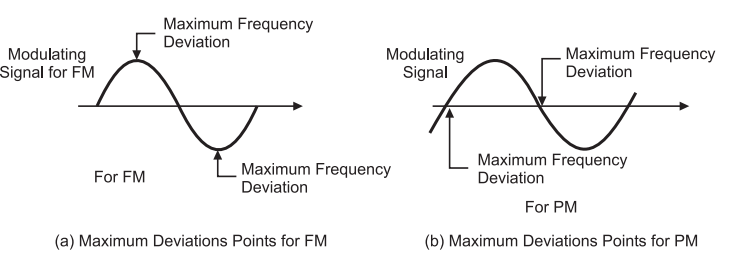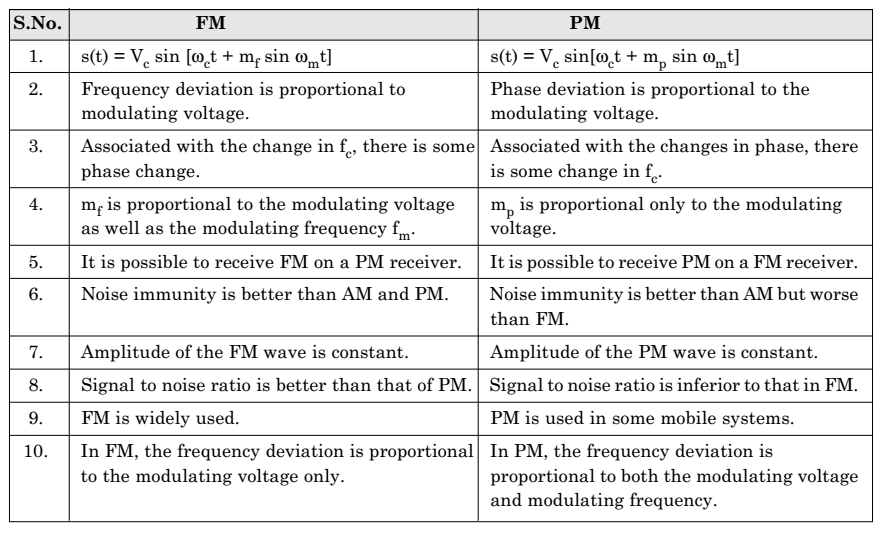# Difference between Frequency Modulation and Phase Modulation

### Difference between Frequency Modulation and Phase Modulation

In this article we are going to understand the difference between FM and PM.

As we already know, phase modulation produces frequency modulation. But, a very important point may be noted here that the frequency modulation (FM) is produced by PM if and only if the phase shift is being varied.

Let us understand this point by considering figure 1 below.Fig. 1: Illustration of difference between FM and PM

### 1.Frequency variation in FM and PM waves

To understand the difference between FM and PM, we first consider the FM wave.

For FM, the frequency changes with the change in modulating signal value.

It remains constant during interval t2 at its maximum value and during interval t5 at its minimum value.

Now, let us look at the PM wave. The frequency of PM wave changes and only if the magnitude of modulating signal changes.

Thus, during the intervals t1, t3, t4, t6, the frequency of PM wave changes. But during the intervals t2 and t5 the frequency of PM remains constant at the carrier frequency value with some phase shift.

### 2. Maximum frequency deviation

The maximum frequency deviation in FM occurs at the point where the rate of change of modulating signal is maximum that means the maximum frequency corresponding to peak values of modulating signal.

But, in PM, the maximum frequency deviation occurs at the point deviation occurs near the zero crossing points of the modulating signal.Thus, although the phase modulation produce FM, the maximum deviation occurs at different points of a modulating signal.

### 3. Effect of modulating frequency

In FM the frequency deviation is proportional only to the amplitude variation of the modulating signal and it is independent of modulating frequency fm.

But, in PM, as the modulating frequency is changed, the rate of change of modulating voltage changes. This changes the frequency deviation.

Thus, in PM, the frequency deviation increases with increase in modulating frequency fm and decreases with decrease in modulating frequency.

### Performance Comparison of FM and PM Systems